# CBSE Question Paper 2014 class 12 Chemistry

CBSE Question Paper 2014 class 12 Chemistry conducted by Central Board of Secondary Education, New Delhi in the month of March 2014. CBSE previous year question papers with the solution are available in the myCBSEguide mobile app and website. The Best CBSE App for students and teachers is myCBSEguide which provides complete study material and practice papers to CBSE schools in India and abroad.

CBSE Question Paper 2014 class 12 Chemistry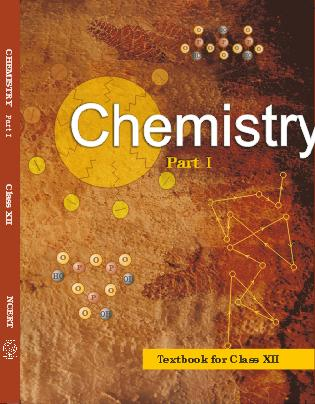## Class 12 Chemistry list of chapters

1. The Solid State
2. Solutions
3. Electrochemistry
4. Chemical Kinetics
5. Surface Chemistry
6. General Principles and Processes of Isolation of Elements
7. The p-Block Elements
8. The d and f Block Elements
9. Coordination Compounds
10. Haloalkanes and Haloarenes
11. Alcohols, Phenols and Ethers
12. Aldehydes, Ketones and Carboxylic Acids
13. Amines
14. Biomolecules
15. Polymers
16. Chemistry in Everyday life

## CBSE Question Paper 2014 class 12 Chemistry

### General Instructions

1. All questions are compulsory.
2. Marks for each question are indicated against it.
3. Question numbers 1 to 8 are very short-answer questions, carrying 1 mark each. Answer these in one word or about one sentence each.
4. Question numbers 9 to 18 are short-answer questions, carrying 2 marks each. Answer these in about 30 words each.
5. Question numbers 19 to 27 are short-answer questions of 3 marks each. Answer these in about 40 words each.
6. Question numbers 28 to 30 are long-answer questions of 5 marks each. Answer these in about 70 words each.
7. Use Log Tables, if necessary Use of calculators is not permitted.

1. Name the depressant which is used to separate ZnS and PbS ores in froth floatation process. 

2. Based on the type of dispersed phase, what type of colloid is micelles? 

3. Arrange the following in the increasing order of their basic character: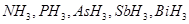4. What type of isomerism is exhibited by the following complex: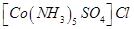5. Write IUPAC name of the following compound: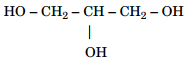6. Which of the two is more basic and why?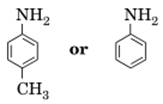7. What are biocatalysts? Give an example. 

8. Express  the  relationship  between  atomic  radius  (r)  and  the  edge length (a) in the b.c.c. unit cell. 

9. Write the role of the following: 

(i)  Iodine in the refining of zirconium

(ii)  Silica in the extraction of copper from copper matte

10. Name the two most important allotropes of sulphur. Which one of the two is  stable  at  room  temperature?  What  happen  when  the  stable  form  is heated above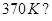Or

(i)  Write  the  conditions  to  maximize  the  yield  of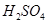by contact process.

(ii) Why is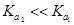forin water? 

11. Complete the following equations: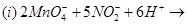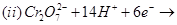12. Write the state  of hybridization, shape and IUPAC name of the complex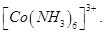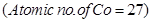13. Write chemical equations when

(i)  ethyl chloride is treated with alcoholic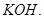(ii)  chlorobenzene is treated with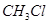in the presence of anhydrous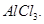14. Write the mechanism of the following reaction: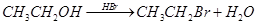15. Name  the  different  reagents  needed  to  perform  the  following reactions: 

(i)  Phenol to Benzene

(ii)  Dehydration of propan-2-ol to propene

(iii)  Friedel − Crafts acetylation of anisole

(iv)  Dehydrogenation of ethanol to ethanol

16. A solution of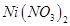is electrolyzed between platinum electrodes using a current of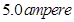for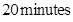. What mass of nickel will be deposited at the cathode?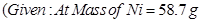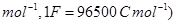17. Define half-life of a reaction. Write the expression of half-life for 

(i) zero order reaction and

(ii) first order reaction.

18. (a) Which alkyl halide from the following pairs would you expect to react more rapidly by an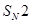mechanism and why?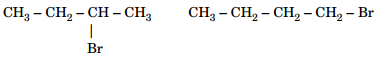(b) Racemisation occurs in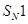reactions. why? 

19. The following data were obtained during the first order thermal decomposition of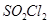at a constant volume: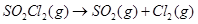Experiment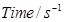Total pressure/atm 1 0 0.4 2 100 0.7

Calculate the rate constant.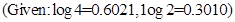20. (i) What type of stoichiometric defect is shown by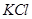and why?

(ii) What type of semiconductor is formed when silicon is doped with As?

(iii) Which one of the following is an example of molecular solid: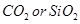(iv) What type of substances would make better magnets, ferromagnetic or ferrimagnetic? 

21. (i) Write two advantages of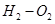fuel cell over ordinary cell.

(ii) Equilibrium constant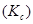for the given cell reaction is 10. Calculate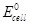.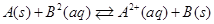22. After the ban on plastic bags, students of one school decided to create awareness among the people about the harmful effects of plastic bags on the environment and the Yamuna river. To make it more impactful, they organized a rally by joining hands with other schools and distributed paper bags to vegetable vendors, shopkeepers and departmental stores. All students pledged not to use polythene bags in future to save the Yamuna river. 

(i) What values are shown by the students?

(ii) What are biodegradable polymers? Give one example.

(iii) Is polythene a homopolymer or copolymer?

23. (i) Define Antihistamine with an example.

(ii) Which one of the following drugs is an antibiotic:

Morphine, Equanil, Chloramphenicol, Aspirin.

(iii) Why is use of aspartame limited to cold food and drink? 

24. Define the following terms: 

(a)  Invert sugar

(b)  Vitamins

(c)  Nucleoside

25. (a) What are the different oxidation states exhibited by the lanthanoids?

(b) Write two characteristics of the transition elements.

(c) Which of the 3d-block elements may not be regarded as the transition elements and why? 

OR

Assign suitable reasons for the following:

(a) The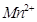compounds are more stable than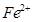towards oxidation to their +3 state.

(b) In the 3d series from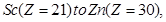the enthalpy of atomization of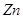is the lowest.

(c)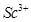is colourless in aqueous solution whereas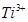is coloured. 

26. (a) What are the different oxidation states exhibited by the lanthanoids?

(b) Write two characteristics of the transition elements.

(c) Which of the 3d-block elements may not be regarded as the transition elements and why? 

OR

Assign suitable reasons for the following:

(a) Thecompounds are more stable thantowards oxidation to their +3 state.

(b) In the 3d series fromthe enthalpy of atomization ofis the lowest.

(c)is colourless in aqueous solution whereasis coloured. 

26. (a) Write the expression for the Freundlich adsorption isotherm for the adsorption of gases on solids, in the form of an equation.

(b) What are the dispersed phase and dispersion medium of butter?

(c) A delta is formed at the meeting place of sea and river water. Why? 

27. Give the structures of A, B and C in the following reactions: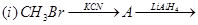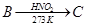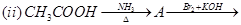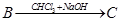28. (a) Account for the following:

(i)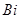is strong oxidizing agent in the + 5 state.

(ii)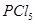is known but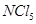is not known.

(iii) Iron dissolves in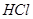to form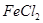and not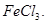(b) Draw the structures of the following: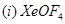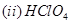Or

(a) Draw the structures of the following: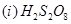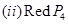(b) Account for the following:

(i) Sulphur in vapour state exhibits paramagnetism.

(ii) Unlike xenon, no distinct chemical compound of helium is known.

(iii)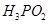is a stronger reducing agent than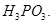29. (a) Write the products formed when ethanal reacts with the following reagents: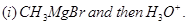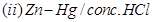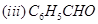in the presence of dilute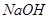.

(b) Give simple chemical tests to distinguish between the following pairs of compounds:

(i) Benzoic acid and Ethyl benzoate

(ii) Propanal and Butan-2-one 

OR

(a) Account for the following:

(i)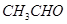is more reactive than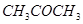towards reaction with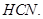(ii) There are two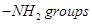in semicarbazide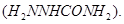However, only on eis involved in the formation of semicarbazone.

(b) Write the chemical equation to illustrate each of the following name reactions:

(i) Rosenmund reduction

(ii) Hell-Volhard-Zelinsky reaction

(iii) Cannizzaro reaction 

30. (a) State Raoult’s law for a solution containing volatile components. Name the solution which follows Raoult’s law at all concentrations and temperatures. 

(b) Calculate the boiling point elevation for a solution prepared by adding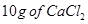to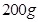of water.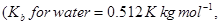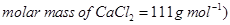Or

(a) Define the following terms: 

(i) Azeotrope

(ii) Osmotic pressure

(iii) Colligative properties

(b) Calculate the molarity of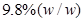solution ofif the density of the solution is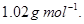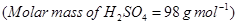These are questions only. To view and download complete question paper with solution install myCBSEguide App from google play store or login to our student dashboard.

## Chemistry Question Paper 2014

Download class 12 Chemistry question paper with solution from best CBSE App the myCBSEguide. CBSE class 12 Chemistry question paper 2014 in PDF format with solution will help you to understand the latest question paper pattern and marking scheme of the CBSE board examination. You will get to know the difficulty level of the question paper. CBSE question papers 2014 for class 12 Chemistry have 30 questions with solution.

## Previous Year Question Paper for class 12 in PDF

CBSE question papers 2018, 2017, 2016, 2015, 2014, 2013, 2012, 2011, 2010, 209, 2008, 2007, 2006, 2005 and so on for all the subjects are available under this download link. Practicing real question paper certainly helps students to get confidence and improve performance in weak areas.

To download CBSE Question Paper 2014 class 12 Accountancy, Chemistry, Physics, History, Political Science, Economics, Geography, Computer Science, Home Science, Accountancy, Business Studies and Home Science; do check myCBSEguide app or website. myCBSEguide provides sample papers with solution, test papers for chapter-wise practice, NCERT solutions, NCERT Exemplar solutions, quick revision notes for ready reference, CBSE guess papers and CBSE important question papers. Sample Paper all are made available through the best app for CBSE students and myCBSEguide website.### Test Generator

Create question paper PDF and online tests with your own name & logo in minutes.### myCBSEguide

Question Bank, Mock Tests, Exam Papers, NCERT Solutions, Sample Papers, Notes